## Example Questions

### Example Question #63 : Quadrilaterals

Quantity A:

The side-length of a square with a perimeter of.

Quantity B:

The side-length of a square with an area of.

The relationship cannot be determined from the information given

Both quantities are equal

Quantity B is greater

Quantity A is greater

Quantity A is greater

Explanation:

The first step to a quantitative comparison is to determine whether it can be solved at all with the given knowledge. Since all you need to find the side-length of a square is the perimeter, the area, OR the diagonal and we have one of each for these two quantities, this relationship can be determined. Thus, "the relationship cannot be determined" is out.

Now, to solve both quantities.  Quantity A can be solved by translating the perimeter into side lengths: the formula for the perimeter of a square is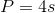, withbeing the side-length, so you just need to divide the perimeter by four.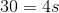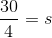Thus, quantity A is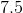.

Quantity B can be solved by translating the area into side lengths: the formula for the area of a square is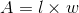, or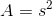, withbeing the side-length, so you just need to find the square root of the area.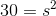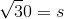Thus, quantity B is roughly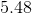.

Therefore quantity A is greater.

### Example Question #64 : Quadrilaterals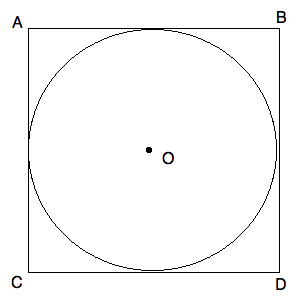Circle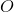has a center in the center of Square.

If the area of Circleis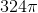, what is the length of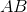?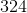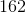Explanation:

If the area of Circleis, we know that the area can be computed using the standard area formula:, usingfor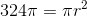Simplifying, we get: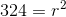We know thatmust be less than. By choosingfor, we find out that this is the radius of our circle. Thus, we know that the diameter of the circle is double this, or. Now, consider the following diagram: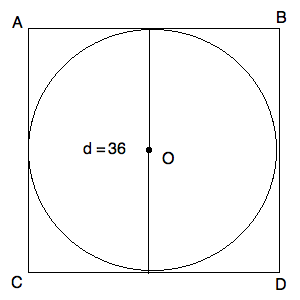Notice that the diameter is the same length as a side of the square. Thus,is equal to.

### Example Question #65 : Quadrilaterals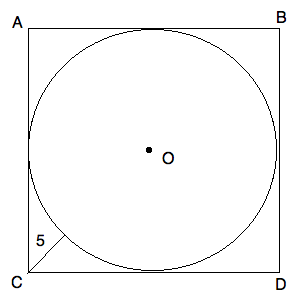Circlehas a center in the center of square.

The line segment marked with lengthlies on the diagonal of the square.

What is the length of side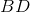?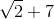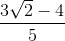This cannot be computed from the given data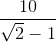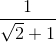Explanation:

You can further fill in your diagram as follows: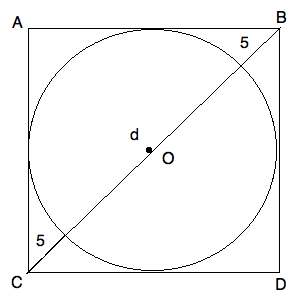Now, we know that the triangle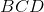is a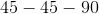triangle. We also know that the length ofand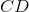must also be equal to the diameter of the circle. (The diameter of the circle will run across the circle horizontally if you draw it that way. This will provide you with a complete side length.) Now, we know that the ratio of the hypotenuse ofto the side must be the same as: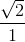For our data, that means: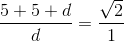Simplifying, we know: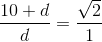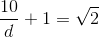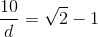Now, make both sides reciprocals: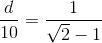Finally, solve: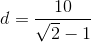Recall, this is both the length of the side and the diameter of the circle. Hence, you have your answer.

Tired of practice problems?

Try live online GRE prep today.